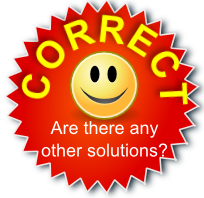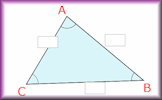Drag four yellow statements into each of the brown rectangles.
Make sure you don't create a paradox!

All of the statements in this rectangle are true

Only three of the statements in this rectangle are true

Only half of the statements in this rectangle are true

Only one of the statements in this rectangle is true

The sum of 8 and 8 is 16

The product of 9 and 12 is 108

The product of 8 and 3 is 24

The product of 5 and 7 is 35

The sum of 12 and 10 is 22

The product of 6 and 4 is 24

The product of 11 and 6 is 72

The product of 12 and 6 is 78

The sum of 3 and 4 is 8

The sum of 3 and 12 is 16

The sum of 11 and 5 is 17

The sum of 7 and 5 is 13## Transum.org

This web site contains over a thousand free mathematical activities for teachers and pupils. Click here to go to the main page which links to all of the resources available.## More Activities:

Mathematicians are not the people who find Maths easy; they are the people who enjoy how mystifying, puzzling and hard it is. Are you a mathematician?

Comment recorded on the 24 May 'Starter of the Day' page by Ruth Seward, Hagley Park Sports College:

"Find the starters wonderful; students enjoy them and often want to use the idea generated by the starter in other parts of the lesson. Keep up the good work"

Comment recorded on the 28 September 'Starter of the Day' page by Malcolm P, Dorset:

"A set of real life savers!!
Keep it up and thank you!"

#### Triangle SolverThis simple calculator will work out the lengths of the sides and the size of the angles of any triangle given thee particular pieces of information.

## Numeracy

"Numeracy is a proficiency which is developed mainly in Mathematics but also in other subjects. It is more than an ability to do basic arithmetic. It involves developing confidence and competence with numbers and measures. It requires understanding of the number system, a repertoire of mathematical techniques, and an inclination and ability to solve quantitative or spatial problems in a range of contexts. Numeracy also demands understanding of the ways in which data are gathered by counting and measuring, and presented in graphs, diagrams, charts and tables."

Secondary National Strategy, Mathematics at key stage 3

## Go Maths

Learning and understanding Mathematics, at every level, requires learner engagement. Mathematics is not a spectator sport. Sometimes traditional teaching fails to actively involve students. One way to address the problem is through the use of interactive activities and this web site provides many of those. The Go Maths main page links to more activities designed for students in upper Secondary/High school.

## Teachers

If you found this activity useful don't forget to record it in your scheme of work or learning management system. The short URL, ready to be copied and pasted, is as follows:

Ktesfai@dallasisd.org,

Sunday, September 30, 2012

"What is the answer to the paradox with the four yellow statements. I can't seem to get it right."

Transum,

Thursday, October 4, 2012

"An example set of answers (there are many different ways of doing this puzzle) are available above for those who have a Transum subscription. Teachers, tutors and parents can apply for a subscription here."

Do you have any comments? It is always useful to receive feedback and helps make this free resource even more useful for those learning Mathematics anywhere in the world. Click here to enter your comments.For All: